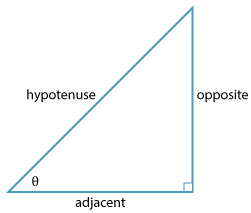There are three basic trigonometric ratios: sine, cosine and tangent, generally shortened to sin, cos and tan respectively.Detailed description

\begin{align}\text{sin} \ \theta &= \dfrac{\text{opposite}}{\text{hypotenuse}}\\ \text{cos} \ \theta &= \dfrac{\text{adjacent}}{\text{hypotenuse}}\\ \text{tan} \ \theta &= \dfrac{\text{opposite}}{\text{adjacent}}\end{align}

A common and easy to remember mnemonic is

S O H C A H T O A

for

Sine – Opposite/Hypotenuse